## 2.101 Introduction

• Variable
• In everyday life, many quantities depend on change in variables:
• Plant's growth depends on sunlight and rainfall.
• A runner's speed == how long it takes to run a distance.

## 2.102 The definition of a function

• Function
• A function is a rule that relates how one quantity depends on another.
• It's central to programming and Computer Science.
• It's a relationship between a set of inputs and a set of outputs, where inputs map to exactly one output.
• Function is a "well-behaved relation"
• Given a starting point, we have just one ending point.
• A function $f$ from a set of $A$ to a set of $B$ is an assignment of exactly one element of $B$ to each element of $A$.
• If $f$ is the function from A to B, we write: $f: A \rightarrow B$
• We can read this as f maps A to B:
• $x \in A: \ \ x \rightarrow f(x) = y \ \ \ (y \in B)$
• Domain of a Function
• Given the function above, $A$ is the set of all inputs and called the "domain" of $f$.
• Written as $D_f = A$
• Co-Domain of Function

• $B$ is the set containing the outputs and called the co-domain of $f$.
• Written as $\text{co-}D_f = B$
• The set of all outputs is called the range of f and is written as $R_f$.
• $y$ is called the image of $x$.
• $x$ is called the pre-image of $y$.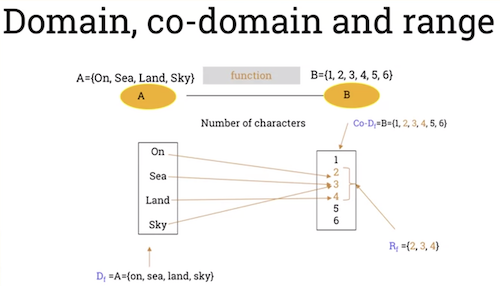• Example: a set mapping characters to a length.

• $f(\text{Sea}) \rightarrow 3$ (contains 3 characters)
• $f(Land) \rightarrow 4$ (contains 4 characters)
• $f(on) \rightarrow 2$
• 2 is the image of "on"
• "on" is the pre-image of 2.
• Conditions under which a relation is not a function:
• Some inputs do not have an image.
• Some inputs have more than one image.
• Exercise 1
• Given the following function: $f: Z \rightarrow Z$ with $f(x) = |x|$, what is domain, co-domain and range for function $f$?
• Domain: $Z$
• Co-domain: $Z$
• Range: $Z^{+}$
• Exercise 2
• Given the following function: $g: R \rightarrow R$ with $g(x) = x^2 + 1$
• Domain: R
• Co-domain: R
• Range: $\{1, 5, 9 ...\}$
• Pre-images(5) = {-2, 2}

## 2.104 Plotting functions

• Linear Function

• Linear function is of form: $f(x) = ax + b$

• Where $a$ and $b$ are real numbers.
• Straight-line function that passes through point (0, b).
• $a$ is the gradient of the function. Where $a > 0$ the function is increasing.
• That is: $x_1 \leq x_2$ then $f(x_1) \leq f(x_2)$.
• Example of increasing linear function: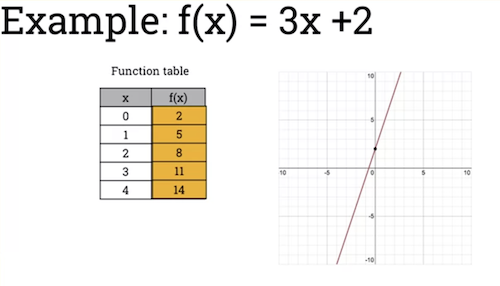• When the gradient is < 0, the function is decreasing.
• $f: R \rightarrow R$
• $f(x) = ax +b$
• If $a > 0$ then function is increasing.
• If $x_1 \leq x_2$ then $f(x_1) \leq f(x_2)$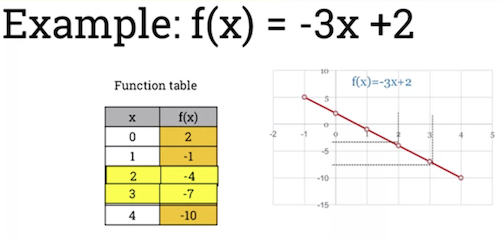• Quadratic functions: $f(x) = ax^2 + bx + c$

• Where $a$, $b$ and $c$ are real numbers and $a \ne 0$.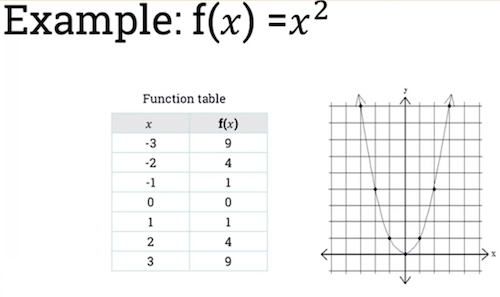• Domain of function f(x) is set of real numbers.

• Range of function is set of positive numbers.
• Exponential Functions
• If base $b$ in $f(x) = b^x$, $b > 1$ then function is increasing and represents growth shown in this graph: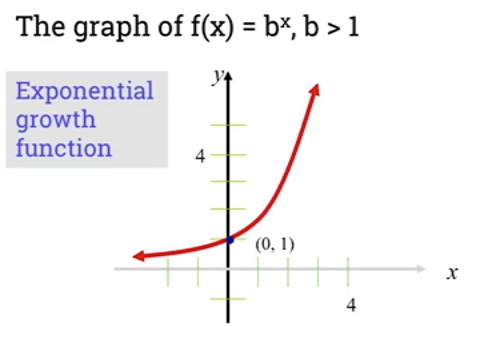* Graph also shows that the point $(0,1)$ is a "common point".
* Domain is equal to set of all real numbers.
* Range is equal to set of all real positive numbers.
* X-axis is horizontal asymtot to curve of function.

• If base 0 < b < 1, then function is decreasing: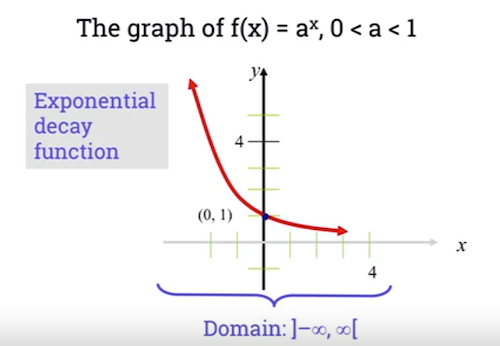• Domain and range are the same as previous function.
• Laws Of Exponential Functions
• $b^xb^y = b^{x + y}$
• $\frac{b^x}{b^y} = b^{x-y}$
• $(b^x)^y = b^{xy}$
• $(ab)^x = a^xb^x$
• $(\frac{a}{b})^x = \frac{a^x}{b^x}$
• $b^{-x} = \frac{1}{b^x}$

## 2.106 Injective and surjective functions

• Injective Function

• A function is considered injective or one-to-one if and only if:

• any 2 distinct inputs will lead to 2 distinct outputs.
• In other words:
• for all $a, b \in A, \text{ if } a \ne b \text{ then } f(a) \ne f(b)$
• same as saying: $a, b \in A, \text{ if } f(a) = f(b) \text{ then } a = b$
• Example on the left is an injective function, as every element of $A$ has a unique image in B.
• Example on the right is not injective. 2 or 4 in A have the same image 0. 1 and 3 have the same image 1.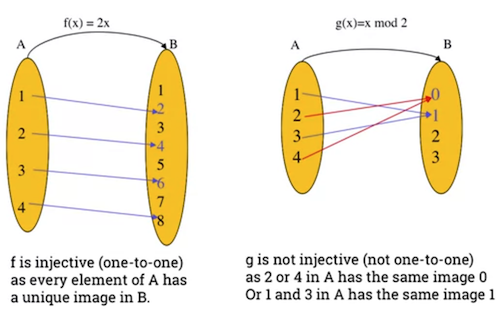• You can show a function is not injective by finding two different inputs $a$ and $b$ with the same Function Image.

• An example with a linear function:
• To show function $f: R -> R$ with $f(x) = 2x + 3$ is an injective function, we must show that $\text{ if } f(a) = f(b) \text{ then } a = b$
• $f(a) = f(b)$ => $2a + 3 = 2b + 3$ => $2a = 2b$ => $a = b$ => f is injective.
• Proof 2:
• Let $a, b \in R$, show that $\text{ if } a \ne b \text{ then } f(a) \ne f(b)$
• $a \ne b$ => $2a \ne 2b$ => $2a + 3 \ne 2b+3$ => $f(a) \ne f(b)$ => f is injective
• An example quadratic function that is not injection.
• Show function $f: R -> R$ with $f(x) = x^2$ is not an injective function
• Example with 2 counter examples that have the same image.
• One example is 5 and -5 have the same image.
• $f(5) = (5)^2 = (-5)^2 = f(-5)$
• Since: $-5 \ne 5$ it's not injective.
• If we change domain to $R^{+}$, the function becomes injective.
• Proof 1:
• Let $a, b \in R^{+}$ show that if $f(a) = f(b)$ then $a = b$.
• Let $a, b \in R^{+}$ show that if $f(a) = f(b)$ then $a = b$
• Proof 2:
• Let $a, b \in R^{+}$ show that if $a \ne b$ then $f(a) \ne f(b)$
• $a \ne b => a^2 \ne b^2$ as $a, b \in R+ => f(a) \ne f(b) => f$ is injective.
• Surjective Function
• A function is said to be a surjective (onto) function if and only if every element of the co-domain of $f$, $B$, has at least one pre-image in the domain of $f, A$.
• In other words, every element in the output domain has some input that will return it.
• for all $y \in B$ there exists $x \in A$ such that $y = f(x)$

• Equivalent to saying range and co-domain of surjective function are the same.
• $\text{ CO}-D_f = R_f$
• Examples: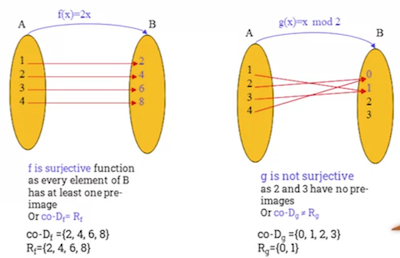• An example Linear Function

• Show that the function $f: R -> R$ with $f(x) = 2x+3$ is a surjective (onto) function.
• Need to show that for any element $y \in R$, there exists $x \in \mathbb{R}$ such that $f(x) = y$
• Proof:
• $f(x) = y$ => $2x + 3 = y$ => $2x = y - 3$ => $x = \frac{y-3}{2} \in R$
• Hence, for all $y \in R$, there exists $x = \frac{y-3}{2} \in R$ such that $f(x) = y$
• An example quadratic function that is not surjective
• Show that function $f: R-> R$ with $f(x) = x^2$ not a surjective (onto) functions
• Proof: * Let $y \in R$, show that there exists $x \in R$ such that $f(x) = y$ * $R_f (\text{ set images }) = [0, + \infty [\ne R(co-D_f) = R$ * We know the range of $Rf$ is positive integers only: all negative images have no pre-images.
• Examples:
• Injective, not surjective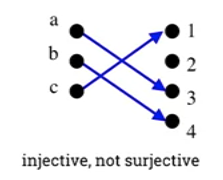• Injective because each element in the domain has a unique image.
• Not surjective because the element 2 in the co-domain has no pre-image.
• Surjective but not injective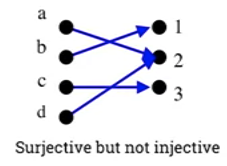• Not injective because a and d are different but have the same image.
• Injective and surjective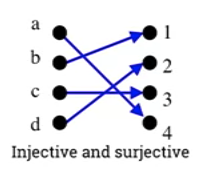• Each element has a unique image.
• Each element in co-domain has at least one pre-image.
• Neither injective nor surjective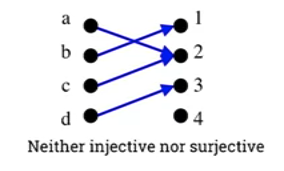• Not injective because a and c have the same image.
• Not surjective because the 4 element of co-domain has no pre-image.
• Not a valid function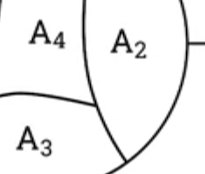• Input a has 2 outputs. In a function, an input can only have a single output.

### Part 1

• *$f_1 : \mathbb{R} \rightarrow \mathbb{R}$ where $f(x) = x^{2} + 1$
• Claim: This function is not injective.
• Proof:
• Let $a = 2$, $b = -2$
• $f(2) = (2)^2 + 1 = 5$
• $f(-2) = (-2)^2 + 1 = 5$
• $f(2) = f(-2)$ therefore the function is not injective.
• Claim: This function is not surjective.
• Proof:
• $f(x) = y$
• $x^2 + 1 = y$
• $x^2 = y - 1$
• $x = \sqrt{(y - 1)}$
• $R \sqrt{(y - 1)} = [1, + \infty [$
• $[1, + \infty [ \ \ne \mathbb{R}$ therefore, this function is not surjective.
• *$f_2 : \mathbb{R} \rightarrow [1, + \infty [ \text{ where } f(x) = x^{2} + 1$
• Claim: This function is not injective.
• Proof:
• Let $a = 2$, $b = -2$
• $f(2) = (2)^2 + 1 = 5$
• $f(-2) = (-2)^2 + 1 = 5$
• $f(2) = f(-2)$ therefore the function is not injective.
• Claim: This function is surjective.
• Proof:
• $f(x) = y$
• $x^2 + 1 = y$
• $x^2 = y - 1$
• $x = \sqrt{(y - 1)}$
• $R _{\sqrt{(y - 1)}} = [1, + \infty [$ therefore, this function is surjective.
• $f_3: \mathbb{R} \rightarrow \mathbb{R} \text{ where } f(x) = x^3$
• Claim: This function is injective.
• Proof:
• $f(a) = f(b)$
• $f(a) = a^3$
• $f(b) = b^3$
• $a^3 = b^3$
• $(a^3)^{1/3} = (b^3)^{1/3}$
• $a = b$ for all $a, b \in \mathbb{R}$ there the function is injective.
• Claim: This function is surjective
• Proof:
• $f(x) = y$
• $x^3 = y$
• $x = \sqrt{y}$
• $\sqrt{y} \in \mathbb{R}$ therefore, the function is surjective.
• $f_4 : \mathbb{R} \rightarrow \mathbb{R} \text{ where } f(x) = 2x + 3$
• Claim: This function is injective.
• Proof:
• $f(a) = f(b)$
• $2a + 3 = 2b + 3$
• $2a = 2b$
• $a = b$ therefore f is injective.
• Claim: This function is surjective.
• Proof:
• $f(x) = y$
• $2x + 3 = y$
• $2x = y - 3$
• $x = \frac{y-3}{2} \in \mathbb{R}$ therefore the function is surjective.
• $f_5: \mathbb{Z} \rightarrow \mathbb{Z} \text{ where } f(x) = 2x + 3$
• Claim: This function is injective.
• Proof:
• $f(a) = f(b)$
• $2a + 3 = 2b + 3$
• $2a = 2b$
• $a = b$ therefore $f$ is injective.
• Claim: This function is not surjective
• Proof:
• $f(a) = 2a + 3$
• $f(x) = y$
• $2x + 3 = y$
• $2x = y - 3$
• $x = \frac{y-3}{2} \notin \mathbb{Z}$ for all $x$ therefore this function is not surjective.

### Part 2

Let $f : \mathbb{R} \rightarrow ]1, +\infty[$ with $f(x) = e^{x} + 1$

1. Show that $f(x)$ is bijection.

• Claim: $f(x)$ is a bijective as it is both injective and surjective.
• Proof of injective:
• $f(a) = f(b)$
• $f(a) = e^a + 1$
• $f(b) = e^b + 1$
• $e^a = e^b$
• $a = b$ therefore the function is injective.
• Proof of surjective:
• $R \ {e^x} = [1, +\infty[$
• $\text{Co-D } e_x = [1, +\infty[$
• $R \ {e^x} = \text{Co-D } e_x$ therefore the function is surjective.
2. Find the inverse function $f^{-1}$.

• $f(x) = y$
• $e^x + 1 = y$
• $e^x = y - 1$
• $x = \log_e(y - 1)$
• $f^{-1}(x) = log_e(x - 1)$
3. Plot the curve of $f$ and $f^{-1}$ in the same graph.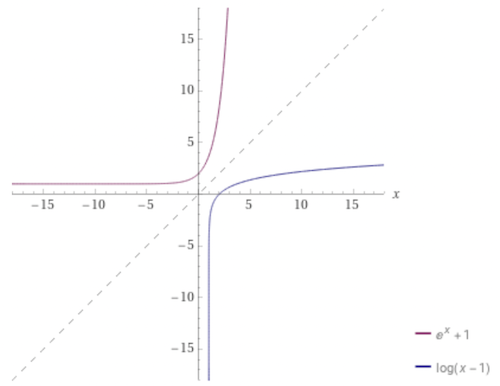4. What can you say about these two curves?

The curves are symmetric with respect to the line $y = x$.